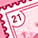# PHP - XML to Array

Announcements

Changes to the LIVEcommunity experience are coming soon... Here's what you need to know.L1 Bithead

## PHP - XML to Array

So I've been working with the API pretty frequently and have run into a bit of a snag that has caught me now a few times.  I'm using CURL with PHP to interact and whenever I get a response, I do a conversion as such:

\$xmlarr = json_decode(json_encode((array)simplexml_load_string(\$result)),1);

This turns the response into a PHP array.  The problem I'm having is that there are certain times when 1 object comes back instead of multiple (querying the traffic log, elements of an object group, etc).  My problem is that when a single object comes back I have to directly query that single object instead of assuming an array is there, which messes up my code.  As an example (filtered for brevity):

```[result] => Array
(
[job] => Array
(
[tenq] => 14:05:39
[tdeq] => 14:05:39
[tlast] => 14:05:42
[status] => FIN
[id] => 10186
)

[log] => Array
(
[logs] => Array
(
[@attributes] => Array
(
[count] => 11
[progress] => 100
)

[entry] => Array
(
 => Array
(
[@attributes] => Array
(
[logid] => 6132182506816674863
)

[src] => x.x.x.x
[dst] => y.y.y.y

 => Array
(
[@attributes] => Array
(
[logid] => 6132182506812719772
)

[src] => x.x.x.x
[dst] => y.y.y.y
```

```  [result] => Array
(
[job] => Array
(
[tenq] => 14:11:05
[tdeq] => 14:11:05
[tlast] => 14:11:07
[status] => FIN
[id] => 10201
)

[log] => Array
(
[logs] => Array
(
[@attributes] => Array
(
[count] => 1
[progress] => 100
)

[entry] => Array
(
[@attributes] => Array
(
[logid] => 6132553591949719329
)

[src] => x.x.x.x
[dst] => y.y.y.y                                )

)

)
```

As you can see - if the log count exceeds is 1, I do not get an array under:

```['result']['log']['logs']
```

this has bit me several times and I'm trying to figure out a good way to fix it.  Any help appreciated!

Tags (3)L4 Transporter

try function load_string down there:

class XmlArray {

public function load_dom (\$xml) {

\$node=simplexml_import_dom(\$xml);

//print "Before node reading\n";

return \$this->add_node(\$node);
}

public function &load_string (\$s) {

\$node=simplexml_load_string(\$s);
//print "Before node reading\n";
\$ret = \$this->add_node(\$node);
return \$ret;
}

private function add_node (\$node, &\$parent=null, \$namespace='', \$recursive=false) {

\$namespaces = \$node->getNameSpaces(true);

\$r['name']=\$node->getName();

//\$content="\$node";
\$content=htmlspecialchars((string) \$node);

if (\$namespace) \$r['namespace']=\$namespace;
if (strlen(\$content)) \$r['content']=\$content;

foreach (\$namespaces as \$pre=>\$ns) {

foreach (\$node->children(\$ns) as \$k=>\$v) {

\$this->add_node(\$v, \$r['children'], \$pre, true);
}

foreach (\$node->attributes(\$ns) as \$k=>\$v) {

\$r['attributes'][\$k]="\$pre:\$v";
}

}

foreach (\$node->children() as \$k=>\$v) {

\$this->add_node(\$v, \$r['children'], '', true);
}

foreach (\$node->attributes() as \$k=>\$v) {

\$r['attributes'][\$k]="\$v";
}

\$parent[]=&\$r;
return \$parent;

}

}L1 Bithead

Thanks for the response - what exactly should be passed into load_string?  The XML response from the API call or?L4 Transporter

string of the API responseL1 Bithead

Yeah unfortunately that ends up creating an array that isn't properly separated..

```\$test = new XmlArray();
\$response = \$test->load_string(\$result);
print_r(\$response);
```

Note: \$result is the response from the Firewall API call.L4 Transporter

Can you show output that you think in incorrect with this version ?

Like what you see?